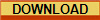Publication History: This article was written especially for "Crain's Petrophysical Handbook" by E. R. Crain, P.Eng in 2015. This webpage version is the copyrighted intellectual property of the author. Do not copy or distribute in any form without explicit permission.Permeability from the LUCIA Method
This is one of the newer permeability methods, designed especially for handling varying pore geometry in carbonates. It was proposed by Jennings and Lucia in "Predicting Permeability from Well Logs in Carbonates", SPE 2003. It is based on a concept called the Rock Fabric Number (RFN) based on the porosity - water saturation relationship in carbonates. Thc Wyllie-Rose method handles the pore geometry problem by using the irreducible water saturation in the denominaror of their equation. The RFN is more explicit.

The relationship between RFN with porosity and saturation, and with permeability and porosity, are shown in the two graphs below.<== Higher water saturation for a given porosity gives higher RFN. Lower permeability for a given porosity gives higher RFN..Rock Fabric Number from Lucia Method
The Lucia method relies on a measure of inter-grain porosity, often callled connected porosity, excluding isolated, vuggy, or moldic porosity. This often considered to be:
1: PHIg = PHIe - PHIsec

Rock Fabric Number (RFN) is derived from:
2: RFN = exp ((7.163 + 1.883 * ln(PHIg) + ln(SWir) ) / (3.063 + 0.610 * ln(PHIg))

Where:
PHIg = inter-grain porosity (fractional)
PHIe = shale corrected porosity from density neutron logs (fractional_
PHIsec = secondary porosity index (SPI) (fractional)
Sw = water saturation (fractional)
RFN = rock fabric number (unitless)

SPL is often assumed to be PHIxdn minus PHIsc, where PHIxdn is shale corrected density neutron crossplat porosity and PHIsc is shale corrected sonic porosity. It may also be assumed to be a constant, a constantPermeability from Lucia Method
3: PERMrfn = exp((27.56 - 12.08 * ln(RFN)) + ((8.671 - 3.603 * ln(RFN)) * ln(PHIg)))

Where:
PERMrfn = permeability (mD)COMMENTS:
You can estimate RFN based on sample descriptions instead of calculating it based on equations 1 and 2. Or use RFN as a calibrating parameter to match core data.RECOMMENDED PARAMETERS:
None required.NUMERICAL EXAMPLE:
SWir = Sw = 0.20
PHIg = 0.20

RFN = exp((7.163 + 1.883 * ln(0.20) + ln(0.20) ) / (3.036 + 0.61 * ln(0.20))) = 3.36
PERMrfn = exp((27.56 - 12.08 * ln(3.36)) + ((8.671 - 3.603 * ln(3.36)) * ln(0.20))) = 400 mDMETA/PERM  Compare Permeability Calculated from Various MethodsPage Views ---- Since 01 Jan 2015